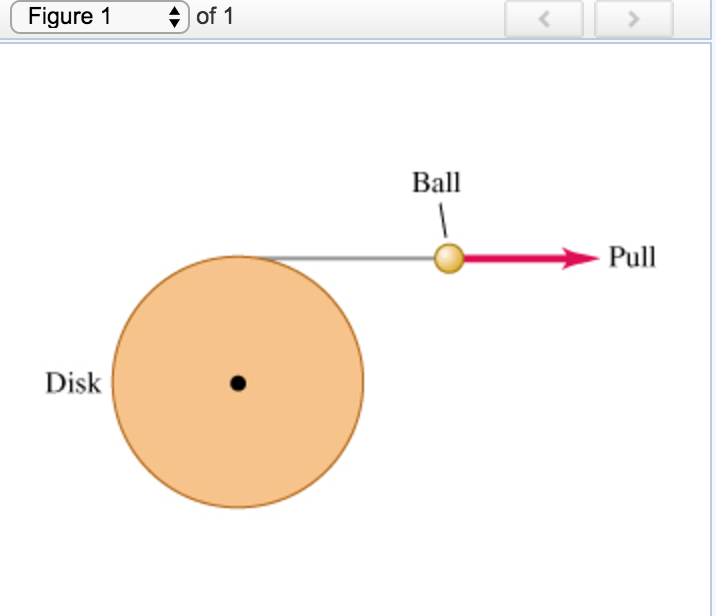# Disk of radius angle problem with part d

A disk of radius 22.0 cm is free to turn about an axle perpendicular to it through its center. It has very thin but strong string wrapped around its rim, and the string is attached to a ball that is pulled tangentially away from the rim of the disk (the figure (Figure 1) ). The pull increases in magnitude and produces an acceleration of the ball that obeys the equation a(t)=At, where t is in seconds and A is a constant. The cylinder starts from rest, and at the end of the third second, the ball's acceleration is 2.20 m/s^2 .a) Find A: (0.733 m/s^3) b) Express the angular acceleration of the disk as a function of time : (3.33t) c) How much time after the disk has begun to turn does it reach an angular speed of 14.0 rad/s ? (2.90 s) d) Through what angle has the disk turned just as it reaches 14.0 rad/s ?

I successfully solved parts a through c (answers in parentheses) but I am unable to solve part d. Help please?